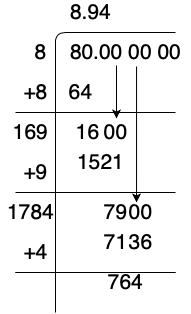Open in App
Not now

# How to find the square root of 80?

• Last Updated : 17 Aug, 2021

Number System is a method of representing Numbers on the Number Line with the help of a set of Symbols and rules. These symbols range from 0-9 and are termed digits. Number System is used to perform mathematical computations ranging from great scientific calculations to calculations like counting the number of Toys for a Kid or Number chocolates remaining in the box.

### Square root

The Square root of a number is that value which when multiplied by itself will give its original number. The square root is represented with the symbol √ called as radical or radix followed by the number which is called radicand i.e., √x.

For example:

√4 = 2

√9 = 3

√16 = 4

√25 = 5

### How to find the square root of 80

There are multiple ways to find the square root of 80, the square root of 80 (√80), or any other number can be found with the help of the following three methods,

• Estimation and Approximation
• Prime Factorization
• Long Division Method

Estimation and Approximation :

The number S is expressed as a * 102n in scientific notation and √S ≈ 6 * 10n , if a >= 10

√80 ≈ 6, with an absolute error of 2.9

Prime Factorization:

• Step 1: Find the prime factors of 80.

80 = (2 × 2 × 2 × 2 × 5)

• Step 2: Pair similar factors.

√80 = √((2 × 2) × (2 × 2) × 5)

√80 = 2 × 2 × √(5)

√80 = 4√5 ≈ 8.94

Long Division Method:

• Step 1: Try to make pairs of 2 digits. From right to left before the decimal point and left to right after decimal points.
• Step 2: Find a number n, such that when multiplied by itself given a number, which is less than or equal to 80. Here, n is 8 as  8 * 8  gives 64. Dividing 80 by 8, we get the quotient as 8 and the remainder as 16.
• Step 3: Drop the next pair down. Here, next is 00 and put it after the remainder of the previous operation which gives us 1600 as a new dividend.
• Step 4: Double the divisor of previous operations, i.e n which gives us 16, and find the largest possible digit(X) such that the quotient for which the product of 16X and X results in a value less than or equal to 1600.
• Step 5: Divide and write the remainder.
• Step 6: Repeat this process according to a given precisionMy Personal Notes arrow_drop_up